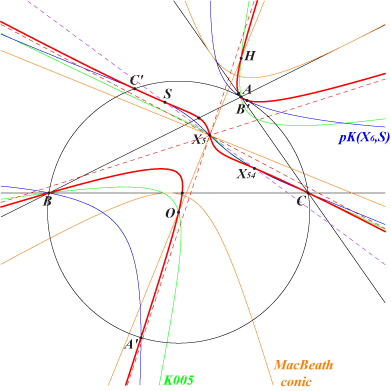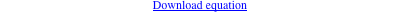too complicated to be written here. Click on the link to download a text file.X(3), X(4), X(5), X(54), X(6288) S = X(6288) = reflection of X(54) in X(5) infinite points of pK(X6, X54) common points of pK(X6, X6288) and the circumcircle foci of the MacBeath conic (O, H and two other imaginary) reflections of A, B, C in X(5) i.e. centers of the Johnson circlesA variable line L passing through X54 is reflected about X5 in L' (passing through X382). The isogonal transform of L meets L' at two points on K526. K526 is a central cubic with center X5, the nine point center. See Table 76. K526 is spK(X54, X5) and its isogonal transform is spK(X6288, X5), two spK cubics as in CL055.